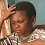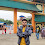# Hackerrank Day 2 Operators 30 days of code solution

In this HackerRank Day 2 Operators 30 days of code problem there is Given the meal price (base cost of a meal), tip percent (the percentage of the meal price being added as a tip), and tax percent (the percentage of the meal price being added as tax) for a meal, find and print the meal's total cost. Round the result to the nearest integer.

## Problem solution in Python 2 programming.

```# Enter your code here. Read input from STDIN. Print output to STDOUT
price = float(raw_input().strip())
tip = int(raw_input().strip())
tax = int(raw_input().strip())
print "The total meal cost is %i dollars." % int(round(price*(100+tip+tax)/100))```

## Problem solution in Python 3 programming.

```#!/bin/python3

import math
import os
import random
import re
import sys

# Complete the solve function below.
def solve(meal_cost, tip_percent, tax_percent):
tip = meal_cost * tip_percent / 100
tax = meal_cost * tax_percent / 100
total_cost = meal_cost + tip + tax

print(round(total_cost))

if __name__ == '__main__':
meal_cost = float(input())

tip_percent = int(input())

tax_percent = int(input())

solve(meal_cost, tip_percent, tax_percent)```

### Problem solution in java programming.

```import java.io.*;
import java.util.*;
import java.text.*;
import java.math.*;
import java.util.regex.*;
public class Arithmetic {

public static void main(String[] args) {
Scanner scan = new Scanner(System.in);
double mealCost = scan.nextDouble(); // original meal price
int tipPercent = scan.nextInt(); // tip percentage
int taxPercent = scan.nextInt(); // tax percentage
scan.close();

double tip = mealCost * tipPercent / 100;
double tax = mealCost * taxPercent / 100;
double total = mealCost + tip + tax;

// cast the result of the rounding operation to an int and save it as totalCost
int totalCost = (int) Math.round(total);

System.out.println("The total meal cost is " + totalCost + " dollars.");
}
}```

### Problem solution in c++ programming.

```#include <cmath>
#include <cstdio>
#include <vector>
#include <iostream>
#include <algorithm>
using namespace std;

int main() {
/* Enter your code here. Read input from STDIN. Print output to STDOUT */
double mealCost;
double totalCost;
int totalCostRound;
int tipPercent;
int taxPercent;

cin >> mealCost >> tipPercent >> taxPercent;
totalCost = mealCost * (1.0 + (double)taxPercent/100.0 + (double)tipPercent/100.0);
totalCostRound = (int)(totalCost + 0.5);
//    cout << mealCost << ", " << tipPercent << ", " << taxPercent << endl;
cout << "The total meal cost is " << totalCostRound << " dollars.";
return 0;
}```

### Problem solution in c programming.

```#include <stdio.h>
#include <string.h>
#include <math.h>
#include <stdlib.h>

int main() {

/* Enter your code here. Read input from STDIN. Print output to STDOUT */
float mealValue;
int tip;
int tax;
unsigned int total;
/* Enter your code here. Read input from STDIN. Print output to STDOUT */
scanf("%f",(float *)&mealValue);
scanf("%d",&tip);
scanf("%d",&tax);

total = mealValue + (mealValue*((float)tip/100)) + (mealValue*((float)tax/100)) + .5;
printf("The total meal cost is %d dollars.", (int)total);
return 0;
}```

### Problem solution in Javascript programming.

```function processData(input) {
var pieces = input.split('\n');
var mealCost = parseFloat(pieces);
var tipPercent = parseInt(pieces);
var taxPercent = parseInt(pieces);

var total = mealCost * tipPercent / 100 + mealCost * taxPercent / 100 + mealCost;

console.log('The total meal cost is ' + Math.round(total) + ' dollars.')
}

process.stdin.resume();
process.stdin.setEncoding("ascii");
_input = "";
process.stdin.on("data", function (input) {
_input += input;
});

process.stdin.on("end", function () {
processData(_input);
});```

1.This comment has been removed by the author.

2.totalCost = mealCost * (1.0 + (double)taxPercent/100.0 + (double)tipPercent/100.0);
totalCostRound = (int)(totalCost + 0.5);

what is the logic behind adding 1.0 in totalCost and 0.5 in totalCostRound ?

1.because we are using double type of variable in taxpercent and tippercent so to convert it into nerest integer we need to add this

2.I did not get your point can u explain some briefly please am new to this please